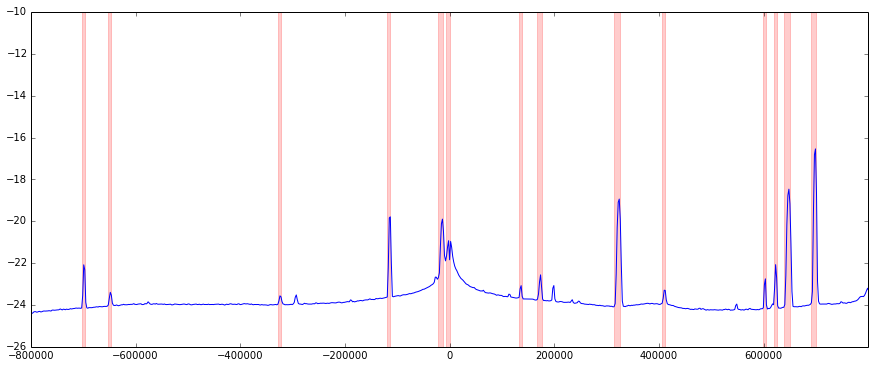In :
%pylab inline
import scipy
import scipy.fftpack
import scipy.signal

import scipy.io

Fc = 463.4e6
Fs = 2e6
N = -1  # Number of samples to pull

pylab.rcParams['figure.figsize'] = (15.0, 6.0)

Populating the interactive namespace from numpy and matplotlib


In :
x = np.fromfile(load_path, dtype="complex64", count=N)

samps = [ 512000, 128000, 1024000, 16000 ]

N = 4
figure(figsize=(15,10))

for i,s in enumerate(samps):
subplot(N/2, 2, i+1)
psd(x[:s], Fs=Fs)
title(s)

None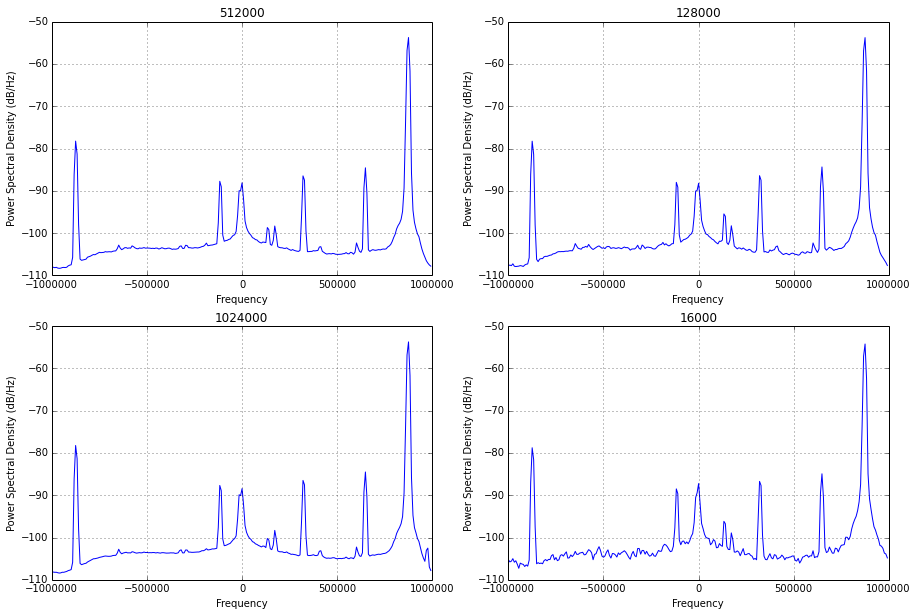In :
samps = [ 16000, 128000, 512000, 1024000 ]
N = len(samps)

figure(figsize=(15,10))

for i,s in enumerate(samps):
subplot(N/2, 2, i+1)
fs, b = scipy.signal.welch(x[0:s], fs=Fs, nperseg=1024)

fs = scipy.fftpack.fftshift(fs)
b = scipy.fftpack.fftshift(b)
plot(fs, np.log(b))
xlim(-Fs/2, Fs/2)
title(s)
None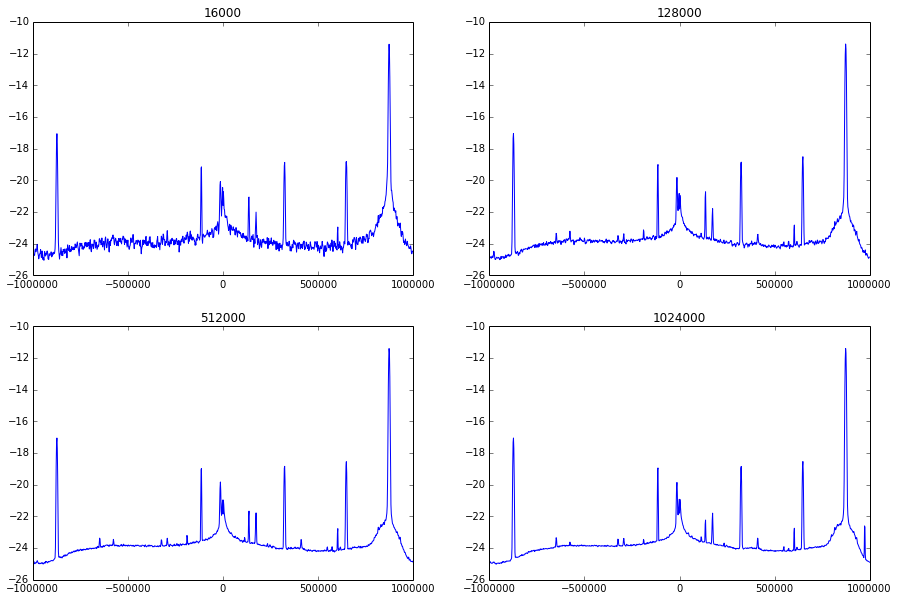In :
fs, b = scipy.signal.welch(x, fs=Fs, nperseg=1024)

fs = scipy.fftpack.fftshift(fs)
b = scipy.fftpack.fftshift(b)

bl = np.log(b)

lpf = scipy.signal.remez(40, [0, 0.001, 0.005, 0.5], [1, 0])
bp = scipy.signal.lfilter(lpf, 1.0, bl)
plot(bp, linewidth = 2)
#ylim(-30, -10)
figure()
plot((bl - bp)[50:])

Out:
[<matplotlib.lines.Line2D at 0x7f5052727e10>]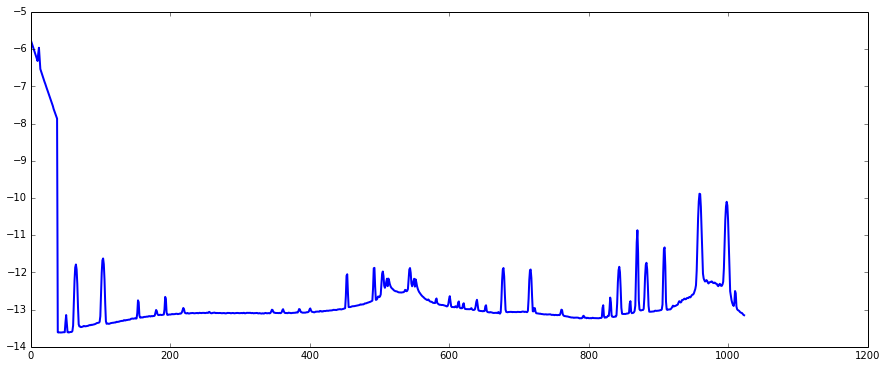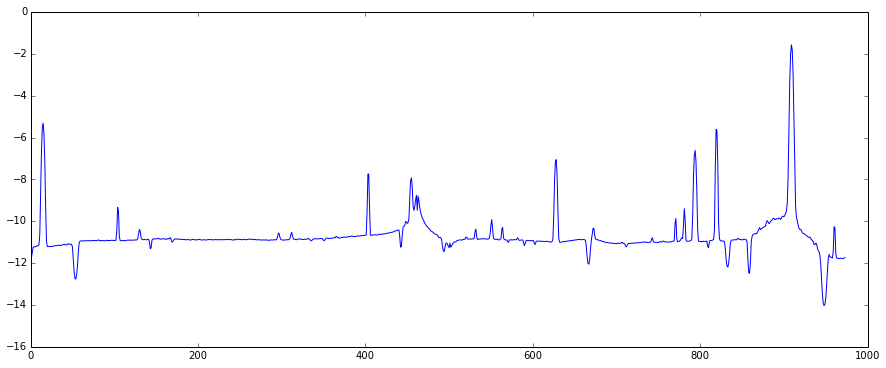Okay, trying to remove the floor with averaging looks like a losing proposition at this point. I could probably better align the two datasets, but let's see what straight-up diffs look like.

In :
plot(diff(np.log(b)))
plot((np.log(b) + 30)/5 )

Out:
[<matplotlib.lines.Line2D at 0x7f50528d7190>]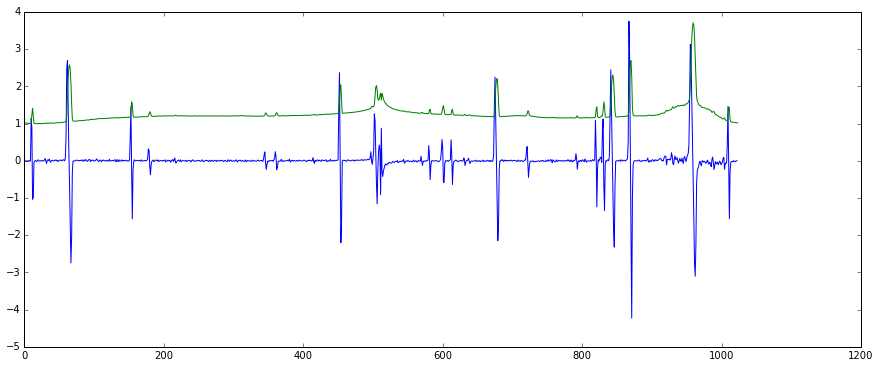In :
hist(diff(np.log(b)), bins=100); None
bl = np.log(b)
dbl = diff(bl)

[ f(dbl) for f in [ np.mean, np.std] ]

Out:
[9.9494280837343398e-06, 0.45431936276595652]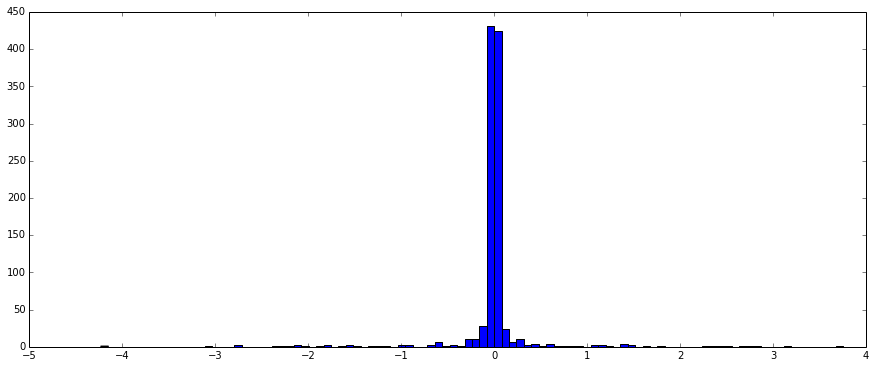In :
plot(dbl)

axhline(np.mean(dbl) + np.std(dbl)*1.5)
None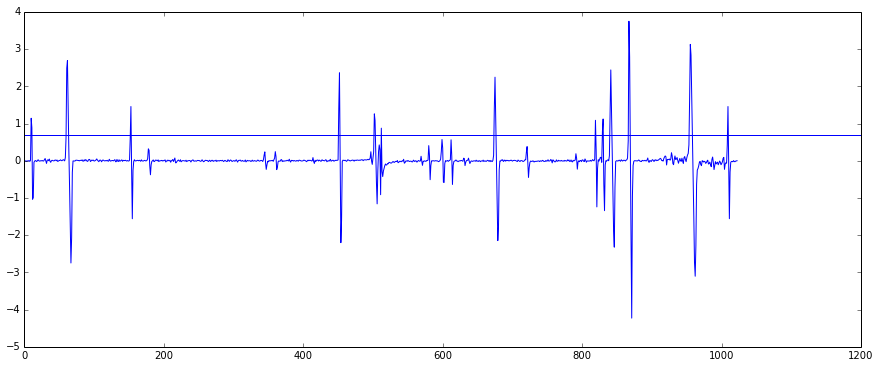This misses a bunch of stuff,because we have a bunch of outliers. Let's focus on the IQR, maybe?

In :
y = sorted(dbl)

[ y[i*len(y)/100] for i in [10, 25, 50, 75, 90] ]

Out:
[-0.056663571687316505,
-0.01390518385232653,
0.00060436665720686733,
0.015055113845683366,
0.047803733896504497]

In :
il = 5*len(y)/100
ih = 95*len(y)/100
yp = y[il:ih]

[ f(yp) for f in [ np.mean, np.std] ]

Out:
[-0.00016012189559754398, 0.042385064050656368]

In :
plot(dbl)

my_floor = np.mean(yp) + np.std(yp)*3.0

axhline(my_floor)

Out:
<matplotlib.lines.Line2D at 0x7f507803e890>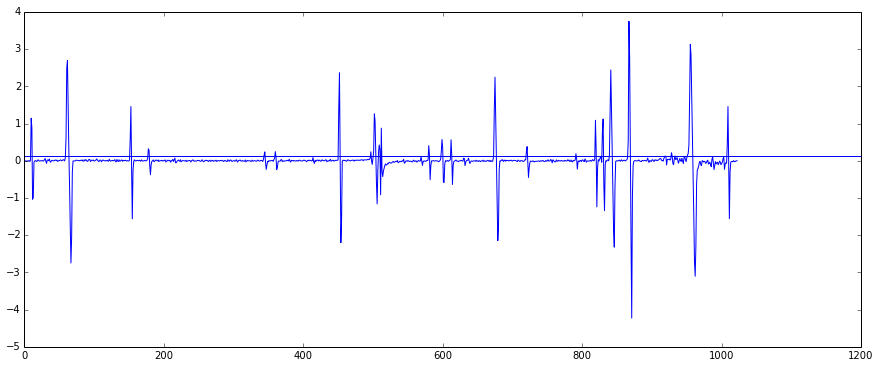In :
figure(figsize=(15,8))

plot(fs, bl)

hits = (np.array(dbl) > my_floor).nonzero()+1
plot(fs[hits], bl[hits], 'o')
xlim(-Fs/2, Fs/2)

Out:
(-1000000.0, 1000000.0)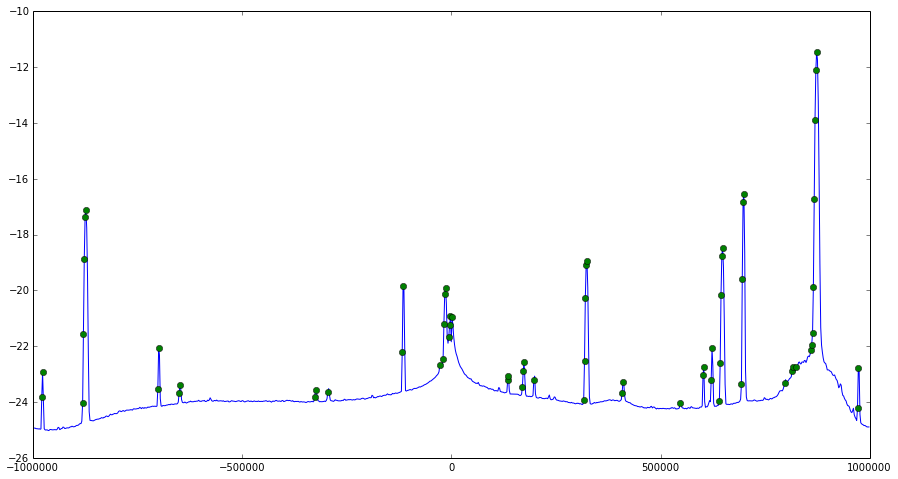Okay, I like this heuristic. Now to figure out channel widths. Let's ignore the top and bottom 10% of the band for now.

In :
hits = hits[ (hits > len(bl)/10) & (hits < len(bl)*9/10) ]

In :
# Do some janky RLEish work to find contiguous channels.
# There are better numpy-ish ways to do this, but this is legible.
runs = []

last_start = None

gap_allowed = 2 # Allow some fudge factor here

for (h0,h1) in zip(hits[:-1], hits[1:]):
if h1 - h0 < gap_allowed:
if None == last_start:
last_start = h0
else:
if None != last_start:
runs.append( (last_start, h0) )
last_start = None

plot(fs, bl)
for h0,h1 in runs:
axvspan(fs[h0-1], fs[h1+1], color="red", alpha=0.2)
xlim(fs[len(bl)/10], fs[9*len(bl)/10])
[ ((fs[h0]+fs[h1])/2, fs[h1]-fs[h0], fs[h0], fs[h1]) for (h0,h1) in runs ]

Out:
[(-700195.3125, 1953.125, -701171.875, -699218.75),
(-649414.0625, 1953.125, -650390.625, -648437.5),
(-325195.3125, 1953.125, -326171.875, -324218.75),
(-116210.9375, 1953.125, -117187.5, -115234.375),
(-16601.5625, 5859.375, -19531.25, -13671.875),
(-3906.25, 3906.25, -5859.375, -1953.125),
(135742.1875, 1953.125, 134765.625, 136718.75),
(171875.0, 3906.25, 169921.875, 173828.125),
(320312.5, 7812.5, 316406.25, 324218.75),
(409179.6875, 1953.125, 408203.125, 410156.25),
(602539.0625, 1953.125, 601562.5, 603515.625),
(622070.3125, 1953.125, 621093.75, 623046.875),
(644531.25, 7812.5, 640625.0, 648437.5),
(696289.0625, 5859.375, 693359.375, 699218.75)]